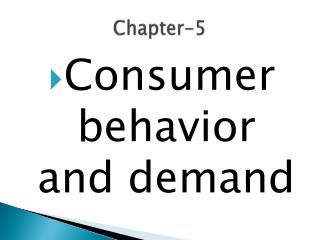DownloadDownload PresentationChapter-5

# Chapter-5

Download Presentation## Chapter-5

- - - - - - - - - - - - - - - - - - - - - - - - - - - E N D - - - - - - - - - - - - - - - - - - - - - - - - - - -
##### Presentation Transcript

1. Chapter-5 • Consumer behavior and demand

2. Utility • Satisfaction, • happiness, • benefit

3. Cardinal Utility vs. Ordinal Utility • Cardinal Utility: Assigning numerical values to the amount of satisfaction • Ordinal Utility: Not assigning numerical values to the amount of satisfaction but indicating the order of preferences, that is, what is preferred to what

4. Ordinal Utility 2 U U x1 0 x2

5. Total Utility The amount of satisfaction obtained by consuming specified amounts of a product per period of time. 5 units of the product per period of time yields 55 utils of satisfaction

6. Marginal Utility The change in total utility (TU) resulting from a one unit change in consumption (X). MU = TU/ X

7. TU, in general, increases with Q At some point, TU can start falling with Q If TU is increasing, MU > 0 From Q = 1 onwards, MU is declining  principle of diminishing marginal utility  As more and more of a good are consumed, the process of consumption will (at some point) yield smaller and smaller additions to utility Total utility and marginal utility Example (Table 4.1): Example (Table 4.1):

8. Total Utility Curve TU 35 30 Figure 4.1 25 Total utility(in utils) 20 15 10 5 Q 0 1 2 3 4 5 6 Quantity

9. Marginal Utility Curve MU 20 15 Marginal utility (in utils) 10 5 0 Q 1 2 3 4 5 6 -5 Quantity Figure 4.2

10. Diminishing Marginal Utility Each additional unit of a product contributes less extra utility than the previous unit. MU = dTU/dX

11. Law of Diminishing Marginal Utility • Example • If I’m really hungry, I get a lot of satisfaction from first slice of pizza. • If I keep eating pizza, the satisfaction from the 8th slice would be much less than that of the first slice.

12. Law of Diminishing Marginal Utility Explanation: As more and more quantity of a commodity is consumed, the intensity if desire decreases and also the utility derived from the additional unit. Assumptions: • All the units of a commodity must be same in all respects • The unit of the good must be standard • There should be no change in taste during the process of consumption • There must be continuity in consumption • There should be no change in the price of the substitute goods

13. Law of Diminishing MU Notes about the Law of Diminishing MU • Time period must be specified for law. • Law tells us that eventually the marginal utility curve will be downward sloping. • Law tells us that eventually the total utility curve will become “flatter.”

14. Marginal Utility MU MU Q

15. Shape of MU • Eventually downward sloping • Law of diminishing marginal utility • Positive always • Rational behavior • Consumer only purchases a good if they get some positive utility from it.

16. Total Utility TU TU DTU DQ DTU DQ Q

17. Shape of TU • Positive slope • Consumer only purchases a good if gets some positive amount of utility (rational behavior) • Slope gets flatter as Q increases • Law of diminishing marginal utility

18. TU, in general, increases with Q At some point, TU can start falling with Q If TU is increasing, MU > 0 From Q = 1 onwards, MU is declining  principle of diminishing marginal utility  As more and more of a good are consumed, the process of consumption will (at some point) yield smaller and smaller additions to utility Total utility and marginal utility Example (Table 4.1): Example (Table 4.1):

19. Graphs of Total Utility & Marginal Utility Total Utility TU X1 is where marginal utility reaches its maximum. This is where we encounter diminishing marginal utility. The slope of TU has reached its maximum; TU has an inflection point here. X X1 X2 Marginal Utility X2 is where total utility reaches its maximum. MU is zero. This is the saturation point or satiation point. After that point, TU falls and MU is negative. MU X1 X2 X

20. Law of Equi-Marginal Utility This law states that the consumer maximizing his total utility will allocate his income among various commodities in such a way that his marginal utility of the last rupee spent on each commodity is equal. Or The consumer will spend his money income on different goods in such a way that marginal utility of each good is proportional to its price

21. Limitations of Law of Equi-Marginal Utility # It is difficult for the consumer to know the marginal utilities from different commodities because utility cannot be measured. # Consumer are ignorant and therefore are not in a position to arrive at an equilibrium. # It does not apply to indivisible and inexpensive commodity.